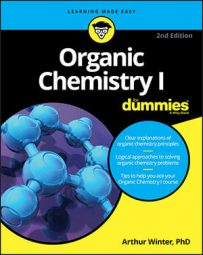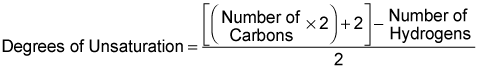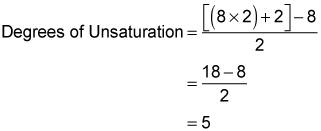##### Organic Chemistry I For DummiesWhen you want to identify the structure of a molecular compound, your first step is to determine the degrees of unsaturation from its molecular formula. This gives a quick indication of whether the compound contains rings, double bonds, or triple bonds, or whether the molecule is saturated.

This is only the first step in finding the molecular structure. You can use the degrees of unsaturation with additional information that you derive from the IR spectrum and the 1H NMR to determine all the fragments the molecule contains. You then put all the fragments together in a way that's consistent with the chemical shift and peak splitting.

You begin by plugging the formula into the equation:If you have a formula with more than just carbons and hydrogens, you also need the following conversions:

• Halogens (F, Cl, Br, I): Add one hydrogen to the molecular formula for each halogen present.

• Nitrogen: Subtract one hydrogen for each nitrogen present.

• Oxygen or sulfur: Ignore.

For example, try plugging the molecular formula, C8H8O2, into the equation:Ignoring the oxygen, you see that C8H8O2 has five degrees of unsaturation.

The degree of unsaturation is valuable information because it tells you how many double bonds, triple bonds, or rings must be present in the unknown compound (although it doesn't tell you which of these elements of unsaturation are present).

Double bonds and rings count for one degree of unsaturation each, and triple bonds count for two degrees each. If the molecule has zero degrees of unsaturation, you know that there are no double bonds, triple bonds, or rings in the molecule.